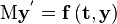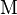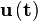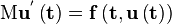# User:Karthik0112358

(Redirected from C S Karthik)

C S Karthik is an undergraduate at IIT-Bombay and is working on adding the Mass Matrix functionality in Radau5 Solver for ASCEND during GSOC2011.

Code branch: karthik:

## Goals

1. Evaluation of mass matrix for a wide range of systems of equations, in the form of a set of CUnit test cases:
1. Accepting all equations and creating a mass matrix of correct dimensions.
2. Identifying all derivatives in an equation.
1. Finding the real values of derivative variables.
2. Identifying the derivatives using these real values.
3. Checking if the equation is linear with respect to the derivatives.
1. If the coefficient of the derivative contains another variable which is not fixed.
2. If the derivative is raised to a power other than 1.
3. If the derivative is in the denominator.
4. If the derivative is present in the index of any term.
5. If the derivative is inside a function.
6. If the coefficient of derivative contains a function.
7. Display corresponding error messages.
4. Evaluating the coefficient.
1. Evaluating a base coefficient to the derivative.
2. Compounding the base coefficient while processing terms in the equation.
3. Processing the coefficients and entering them to the mass matrix.
5. Test with CUnit.
2. Complete DAE solution of those solutions using RADAU5.

## Progress reports

August 24

Example of mass matrix for DAE.

August 22

August 19

Wrote test_radau5.c. Working on some issues.

August 15

• CUnit up and running.
• Code completed for writing mass matrix, adding comments and checking if I missed any cases. Only integrating to Radau5 left.

August 4

I have completed writing the function which evaluates the coefficient after confirming the equation is linear with respect to the derivatives. Code: Coefficient_Calculator(). Hence Goal 1.4 complete.

July 31

July 25

Was not able to commit to svn due to current LAN problems at college. Will commit tomorrow. For now here is the code: Coefficient_Calculator().

July 14

Wrote code for catching errors in equation where the derivative is raised to a higher/lower power. This was part of Goal 1.3.2. Svn committed the code: http://code.ascend4.org/viewvc/code/branches/karthik/ascend/compiler/coefficient_calculate.c .

July 13

I have come up with a simplified algorithm for Goal 1.4.2 after going through the code which simplifies equations. Will try to implement it.

July 12

I have decided to get the job done in two parts. First a function called Inspect_Equation, which determines if the equation can be solved by Radau5 and is linear. Once the given set of equations pass this function, the coefficients are evaluated in the coefficient_match function.

July 6

I have some svn commits:

One more function in progress. Will add that too ASAP.

July 1

• I just found out that during execution, the relations are stored in a simplified manner. As in if we have an equation:

(20+9)*6*dx_dt= ((3.0+1)*dx_dt) + 4*x + 7/x

Then it gets reprocessed as:

174*dx_dt= 4.0*dx_dt + 4*x + 7/x

So, Evaluation of coefficient is solved pre-hand. I do not need to worry about this. The equations are simplified and stored in relation structure.

• So currently I am looking at a way to implement this piece of code, I had written a few days back: Classify_Var(). I need to tweak it into Coefficient_Calculator() so that I can identify if a term has a derivative. They rest is already done.
• John Pye suggested I concentrate only on linear equations in y'. This reduces my job to give errors in every other case. I am thinking of trying to read if the coefficients of derivatives are either algebraic variables or constants. Otherwise I will display an error message for now. John Pye asked me to display helpful messages along with errors. I will work on that right at the next stage.

June 30

Implementation of the pseudo-code: Coefficient_Calculator().

June 29

So I am updating here some Pseudo-Code regarding the approach at the lowest level:

1. So the code for reading each equation and extracting each term is written in Coefficient_Calculator(). So I start here at the point where I have each term.
2. I write a switch case which considers cases based on the type of the relation term. However each term processed is stored in an structure in a particular way. All numbers are stored in an floating point array, all variables in a character array, all operators in an enum and and array to maintain the order of entry of these terms.
1. Its a real number
2. If its a variable, then:
1. If identified as a term containing a derivative, then I evaluate the coefficient the following way:
1. Since we have the relation evaluated in postfix manner, I run backwards in the array which contains the order, to find the last collection of terms which makes sense, as in numbers separated properly by operator, etc. I evaluate this to be the coefficient.
3. Its an operator
3. After evaluating the entire relation, if a particular derivative was not found, then by default it will be assigned a coefficient of 0.

Again this was the basic outline. After discussing with John Pye, he suggested to consider more cases and I will build on the above pseudo-code.

June 28

So what am I able to code uptill now? The following have been accomplished:

1. I am able to read each equation independently.
2. I am able to segregate each term. I am able to identify them as numbers, operators or variables.
3. I am able to find an index for all variables. The algebraic come first followed by the differential.

What are the problems to be addressed?

1. I am able to capture coefficients of all the terms. But how do I separate the differential terms from the algebraic ones? If only there was a way I knew to find the number of differential or algebraic variables involved (ascend/integrator has some code which addresses this. But it uses variable_var structure).

June 25

Some more doxy searches. I am assuming code written on June 24 to be correct and proceed with exploiting var_variable structure.

So here is an idea. To understand the working of relation_term structure, I add print statements in doxy:RelationEvaluateResidualGradientSafe() and try to see how the data members interact.

Apparently, Expr_enum is there for abstraction or atleast it seems so. I do not see any other way out other than the code I wrote yesterday. Need some suggestion here. As a last resort in case John Pye does not agree with my previous idea, I will have to brute force every equation into a particular way by moving terms across (which John Pye did not like).

June 24

I am writing skeletal code for Goal Part 1.1 and 1.2. Will remove it if John Pye tells me it is not apt.

So I have added some code here: Coefficient_Calculator().

Further I have written a function needed for above code here: Classify_Var().

I went through a lot of doxy pages, but I was unable to find a way by which I could take data from relation_term structure's term and pass it to Classify_Var() which accepts var_variable structure. I need some assistance here. If I am able to find a way then Goals: 1.1 and 1.2 would be over.

PS: All function references used in Coefficient_Calculator() and Classify_Var() are either from ascend/compiler or ascend/system and none of ascend/integrator are used.

June 23

Today is more like a "No-Coding-day". I plan to spend the entire day studying all the references John Pye has given me over the last 2 days. Trying to understand expression evaluation data structures to greater depths. Will report more.

1. Implementation of an Ascend Interpreter: Ascend is an object oriented language and hence the concept of dealing with instances. A tree data structure was chosen so that parent-child linkage could be exploited for effective storage and referencing. It is followed by explanation of various functionalities - same, alike, arrays in ASCEND. Base types, Relations are discussed. After Parsing, we finally come to the main topic- Instantiation. There is stress on the fact that ASCEND executes in a line by line fashion. The Multi-Pass Instantiator seems to have solved the problem of the interpreter's capability to allow multiple passes by storage of unexecuted instructions in instance data structure. The explanation provided through an algorithm is excellent demonstration. Final Note: Although gave me nice insight, not useful for resolving problem at hand.

June 21

Finally I have been able to reach the lowest level for coding this mass matrix. It involves rewriting doxy:RelationEvaluateResidualGradientSafe(). The code as stated below requires some understanding. John Pye had provided some references. Will see if they are related and may help me.

Hopefully the last of the skeletal code: Coefficient_Calculator().

So here is some explanation of the intent of Coefficient_Calculator(): I have assumed the equation looks like Q(x,y,y')=0. I have tried to find the length of the relation and get each term out. I have dealt with finishing cases also such as when both lhs and rhs are processed or when pointers are passed to top of stack. I would like to add a switch case for various terms which would be preceded by the following code segment:

term = NewRelationTerm(r, t++, lhs);


June 20

I have worked on a skeletal code (this is an addition to previous skeletal code- in other words providing some flesh to the skeleton) where I try to determine a row entry of the mass matrix: coefficient_find().

Again here I have tried to get a required version of relman_diff2() of ascend/system/relman.c. So in crux the entire task boils down to rewriting RelationCalcResidGradSafe() of ascend/compiler/relation_util.c into a function which gets the coefficients of the derivative terms - Coefficient_Calculator(). I will get a code out for that.

As of now I have a partial code for Coefficient_Calculator():

int Coefficient_Calculator(struct Instance *i){
struct relation *r;
enum Expr_enum reltype;
int dummy_int;
r = (struct relation *)GetInstanceRelation(i, &reltype);
if( r == NULL ) {
ERROR_REPORTER_HERE(ASC_PROG_ERR,"null relation\n");
return -1;
}
//At this point of writing the code, I realize the significance of why John Pye had asked me to understand expression-evaluation data structures. I need to write cases for reltype. I need some thinking.
}


As part of understanding doxy:Expr_enum better, I would like to do a bit of "doxy search". Will get back with some update.

Simultaneously I will also write some test code file in compiler/test/ and try to see if I can get to know the contents of the structure. I will report worthy findings here.

June 19

So here is some skeletal-code: massmatrix(). This is code for one of the top layers.

I know I haven't followed the coding style of Ascend. I will modify code ASAP once other parts fall into place.

June 17

After giving it some thought, I think the structure of the mass matrix function should look more like system_jacobian() which is present in jacobian.c in ascend/system. Will sketch a pseudo code out after better clarity.

I am trying to see how to utilize doxy:System Structure, as I see potential information regarding extracting coefficients of yi' in equation. Since I am asked not to access the structure directly, I am looking through its implementation in slv_client.h.

After reading ascend/system/slv_client.h comments (which I might add are very descriptive and helpful), I realize the importance of the rel_relation structure as this contains the equation data. I have stopped trying to understand struct system and focus back on rel_relation.

So here is a plan. I replicate the entire set of codes required to find the Jacobian matrix. Now from what I know entries to the Jacobian are done row wise. Now Jacobian(mXn matrix) is found out for functions F : RnRm. However with the mass matrix problem, I am dealing with equations. So, my idea is to manipulate these equations into functions and replace the content of the (i,j) entry (by replacing the code which writes the content) into Jacobian with a different set of code, so that we get desirable result. Will get back on this.

June 16

#include <ascend/compiler/rel_blackbox.h>
#include <ascend/compiler/relation.h>
#include <ascend/compiler/relation_util.h>
#include <ascend/compiler/relation_io.h>
#include "mass_matrix.h"
#include "slv_server.h"
#define IPTR(i) ((struct Instance *)(i))
#define KILL 0 /* compile dead code if kill = 1 */
#define REIMPLEMENT 0 /* code that needs to be reimplemented */
#define rel_tmpalloc_array(nelts,type)  \
((nelts) > 0 ? (type *)tmpalloc((nelts)*sizeof(type)) : NULL)
static double dsolve_scratch = 0.0;        /* some workspace */
#define DSOLVE_TOLERANCE 1.0e-08                /* no longer needed */
#define BROKENKIRK 0
/* return 0 on success (derivatives, variables and count are output vars too) */
int massmatrix(struct rel_relation *rel, const var_filter_t *filter
,real64 *derivatives, int32 *variables
,int32 *count){
const struct var_variable **vlist=NULL;
int32 len,c;
int status;
//CONSOLE_DEBUG("In Function: relman_diff2");
assert(rel!=NULL && filter!=NULL);
len = rel_n_incidences(rel);//this gives me the length of incidence- which I think is the number of columns of mass matrix
vlist = rel_incidence_list(rel);//have to write rel_mass_matrix() which basically does the same thing
*count = 0;

for (c=0; c < len; c++) {
//write the stuff here

}
return status;
}


June 15

• Trying to understand about expression-evaluation data structures by reading the following c code: ascend/system/relman.c.
• Re-reading Developer's Manual for better clarity.
• Installing MOOCHO: Downloaded trilinos-10.6.4-Source.tar.gz. As first step of the installation, I downloaded CMake. In the last step of Installation of CMake, I got the following error:

CMake Error at cmake_install.cmake:36 (FILE): file cannot create directory: /usr/local/doc/cmake-2.8. Maybe need administrative privileges.

make: *** [install] Error 1

Further on trying to install Trilinos, with the following commands, I got the following error :

~/SOME_BUILD_DIR$cmake \ > -D CMAKE_BUILD_TYPE:STRING=DEBUG \ > -D Trilinos ENABLE <moocho>:BOOL=ON \ > -D Trilinos_ENABLE_TESTS:BOOL=ON \ > -D DART_TESTING_TIMEOUT:STRING=600 \ >$EXTRA_ARGS \

> {TRILINOS_HOME}

bash: moocho: No such file or directory

I contacted Bartlett, Roscoe A (rabartl@sandia.gov) via email regarding this problem. Hoping for an early reply.

June 6 - June 12

Finding a temporary fix for the mass matrix problem. I have split the task as to finding solution to 2 problems:

• (i) Making sure algebraic equations are being sent to solver.
• (ii) Writing a feasible mass matrix function to generate the mass matrix. This depends on (i).

Regarding Solving Part (i), I made an outside link and was able to capture all the variables involved. Trying to use them to find total number of equations. In completing part (ii) I am facing "INSUFFICIENT MEMORY" problem. Trying to fix that too. I am at present able to count total number of variables, solver variables, independent variables and number of differential equations. I would like to figure out a way to count number of free variables.

I have been able to find a solution to part(i), but it has 2 constraints,

• If number of total variables>>total number of equations, then the max number of substeps has to be reduced.
• The solution involves providing data outside given data structures and thus cannot employed as it is to ascend.

As far as part (ii) goes, there seems to be a simple fix. I am trying to figure it out through Valgrind's Memory loss method.

May 30 - June 5

May 23 - May 29

Prior to 23 May 2011:

Related to rSQP:

• Started reading Mathematical and High-Level Overview of MOOCHO. However was later redirected to chapter 12 (Nonlinear programming) of "Operations Research: Applications and Algorithms" from Winston, 1994, 3rd (or a later) Ed., Duxbury Press (Belmont, California) as a preliminary reading for mathematical background.
• Completed reading chapter 12 (Nonlinear programming) of "Operations Research: Applications and Algorithms".
• Re-reading Mathematical and High-Level Overview of MOOCHO.
• Revisited theory behind the Simplex Algorithm from "Introduction to Algorithms", 3rd Edition, Page 864-879 authored by Cormen, Leisersin, Rivest, Stein.

• Investigated code ascend/integrator/integrator.h and ascend/integrator/integrator.c to understand the data structures used.
• Devised and tried implementation of various for the mass matrix problem in solvers/radau5/asc_radau5.c.
• Settled on the idea of replacing neq with total number of equations as compared to the previous implementation of number of states.

## Mathematical note on my project

• RADAU5 is used to find numerical solutions for a stiff (or differential algebraic) system of first order ordinary differential equations.
• Problems must be of the form$\mathrm{M} \mathbf{y^{'}} = \mathbf{f} \left(\mathbf{t},\mathbf{y} \right)$ with possibly singular matrix$\mathrm{M}$. Any solution to this equation is a function$\mathbf{u} \left(\mathbf{t} \right)$ satisfying$\mathrm{M} \mathbf{u^{'}\left(\mathbf{t} \right)} = \mathbf{f} \left(\mathbf{t},\mathbf{u\left(\mathbf{t} \right)} \right)$.
• An implicit Runge-Kutta method of order 5 (Radau IIA) is implemented to solve problems.
• We refer to$\mathrm{M}$ in$\mathrm{M} \mathbf{y^{'}} = \mathbf{f} \left(\mathbf{t},\mathbf{y} \right)$, as the Mass Matrix for the set of equations.
• If the dimension of the Mass Matrix,$\mathrm{M}$ is axb (i.e. a rows and b columns) then a is equal to the number of equations and b is equal to the dimension of vector space in which y(t) lies.
• Let mij be the element at the mth row and nth column of Mass Matrix$\mathrm{M}$. So mij are the coefficients of the derivative operators in the set of Differential Algebraic equations. Note that every mij has to be a constant.
• Initial data and the set of equations are accepted through ascend model files and the mass matrix is calculated (ONCE).
• The solutions are determined in a neighborhood of the initial input time by RADAU5 solver.